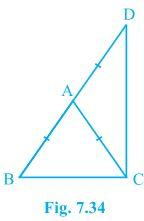# ΔABC is an isosceles triangle in which AB = AC. Side BA is produced to D such that AD = AB (see Fig.) . Show that BCD is a right angle.#### Solution

Given

AB = AC and AD = AB

To Prove

BCD is a right angle

Proof

In ∆ABC,

AB=AC

∠ACB=∠ABC ——————–(i)

In ∆ACD,

On adding equations (i) and (ii) we get

∠BCD=∠ABC+∠BDC

∠BCD+∠BCD=∠ABC+∠BDC+∠BCD

2∠BCD=180°

∠BCD=(180°)/2=90°

Hence Proved(25)(9)